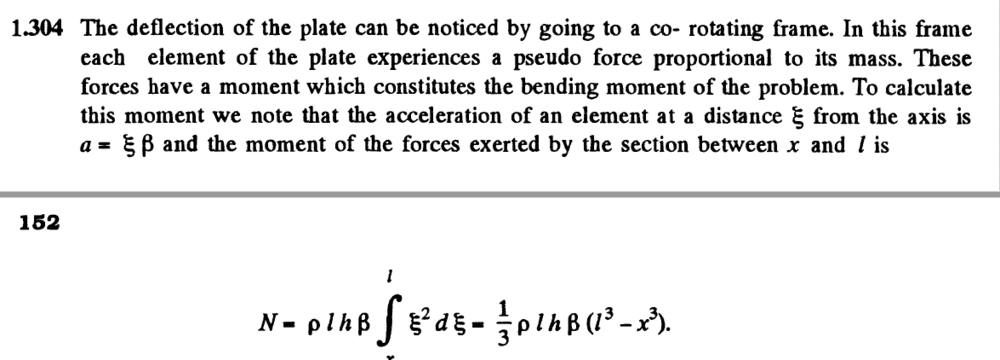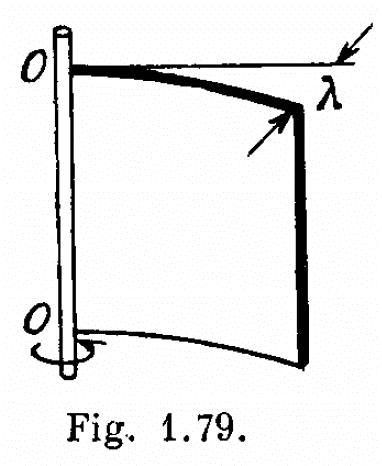# I.E. Irodov Problems in General Physics, 1.304

Luke Tan
Homework Statement:
A steel plate of thickness h has the shape of a square whose side equals l, with h << l. The plate is rigidy fixed to a vertical axle OO which is rotated with a constant angular acceleration ##\beta##. Find the deflection ##\lambda##, assuming the sagging to be small
Relevant Equations:
$$EI\frac{d^2y}{dx^2}=N(x)$$
My struggle here comes from finding the bending moment ##N(x)##. My working is as follows.

We want to find the bending moment on an element a distance ##x## away from the axis of rotation. To do so, let us consider the bending moment due to the force on an element ##\xi>x## away from the axis of rotation. The perpendicular force on this element is ##\xi\beta dm##. Hence, the total bending moment on this element would be
$$N=\int_x^l(\xi-x)(\xi\beta \mu d\xi)$$
Where ##\mu=\rho l h## is the mass per unit length. However, the solutions manual has this expression(Solutions to I.E. Irodov's Problems in General Physics, Volume 1, Second Edition, Singh)

Where the integrand is ##\xi^2##, rather than ##\xi(\xi-x)##. I initially thought that the solutions manual was wrong, but it seems like sources all over the internet have ##\xi^2## under the integral sign. Can someone explain to me what I did wrong?

Thanks!

•etotheipi

Homework Helper
Gold Member
2022 Award
A diagram would help. Is the plate in a horizontal or vertical plane? Where is the axle in relation to the plate?

Luke Tan
A diagram would help. Is the plate in a horizontal or vertical plane? Where is the axle in relation to the plate?
I forgot to attach the given diagram, here it is (the axle is vertical, thus the plate is vertical)#### Attachments

Homework Helper
Gold Member
2022 Award
Then I agree with your integral.

Yes I also agree with yourself and @haruspex. The bending moment ##N(x)## is applied to the element about a vertical axis passing through an arbitrary point on the cross section connecting the element and the rest of the square. The solution they give computes it about the axis OO, which doesn't make sense!

Last edited by a moderator:
•Lnewqban
Just in case anyone's interested, there's another way you could look at the solution that makes it slightly less wrong (but still wrong).

The internal forces acting on the outer element are comprised of a shear force and a bending moment. The easiest way to solve it would be to, as you did, balance moments about the point ##x##, since then the shear force has no moment. However, we could also balance the moments on this element about the axis OO.

The bending moment is, as always, produced by a couple so its moment about the axis OO is exactly the same as that about a parallel axis through the point ##x## - which is what you get if you evaluate your integral. Except now we also need to account for the contribution of the shear force, at a distance ##x## from OO, which we can compute by balancing forces in the axial direction: $$F_s = \int_x^l \xi \beta dm = \int_x^l \xi \beta \rho h l d\xi = \frac{1}{2}\beta \rho h l (l^2 - x^2)$$ $$\tau_s = \frac{1}{2}x\beta \rho h l (l^2 - x^2)$$ You will see that if you do the integral you wrote down, this term ##\tau_s## will be the difference between it and the book answer.

Last edited by a moderator:
•Lnewqban
Homework Helper
Gold Member
What does create the deflection, only the mass of the steel plate or some additional drag force from the fluid surrounding it?
Could you solve the problem as a cantilever beam under uniformly distributed load?

https://www.engineeringtoolbox.com/cantilever-beams-d_1848.html•etotheipi
What does create the deflection, only the mass of the steel plate or some additional drag force from the fluid surrounding it?
Could you solve the problem as a cantilever beam under uniformly distributed load?

https://www.engineeringtoolbox.com/cantilever-beams-d_1848.htmlIn the rotating frame, there's an inertial force proportional to the tangential acceleration ##\beta## that results in a non-uniform loading on the structure. So the cantilever approach is definitely a good one.

You'll see that I have you to thank for post #6•Lnewqban
Homework Helper
Gold Member
In the rotating frame, there's an inertial force proportional to the tangential acceleration ##\beta## that results in a non-uniform loading on the structure. So the cantilever approach is definitely a good one.

You'll see that I have you to thank for post #6Thank you very much for the clarification, etotheipi.In that case, the inertial load is not uniformly distributed, but dependent on the radius.

•etotheipi